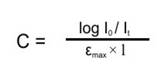# Applications of Absorption Spectroscopy (UV, Visible)

1. Detection of Impurities
UV absorption spectroscopy is one of the best methods for determination of impurities in organic molecules. Additional peaks can be observed due to impurities in the sample and it can be compared with that of standard raw material. By also measuring the absorbance at specific wavelength, the impurities can be detected.
Benzene appears as a common impurity in cyclohexane. Its presence can be easily detected by its absorption at 255 nm.

2. Structure elucidation of organic compounds.
UV spectroscopy is useful in the structure elucidation of organic molecules, the presence or absence of unsaturation, the presence of hetero atoms.
From the location of peaks and combination of peaks, it can be concluded that whether the compound is saturated or unsaturated, hetero atoms are present or not etc.

3. Quantitative analysis
UV absorption spectroscopy can be used for the quantitative determination of compounds that absorb UV radiation. This determination is based on Beer’s law which is as follows.
A = log I0 / It = log 1/ T = – log T = abc = εbc
Where ε is extinction co-efficient, c is concentration, and b is the length of the cell that is used in UV spectrophotometer.
Other methods for quantitative analysis are as follows.
a. calibration curve method
b. simultaneous multicomponent method
c. difference spectrophotometric method
d. derivative spectrophotometric method

4. Qualitative analysis
UVabsorption spectroscopy can characterize those types of compounds which absorbs UV radiation. Identification is done by comparing the absorption spectrum with the spectra of known compounds.
UV absorption spectroscopy is generally used for characterizing aromatic compounds and aromatic olefins.

5. Dissociation constants of acids and bases.
PH = PKa + log [A-] / [HA]
From the above equation, the PKa value can be calculated if the ratio of [A-] / [HA] is known at a particular PH. and the ratio of [A-] / [HA] can be determined spectrophotometrically from the graph plotted between absorbance and wavelength at different PH values.

6. Chemical kinetics
Kinetics of reaction can also be studied using UV spectroscopy. The UV radiation is passed through the reaction cell and the absorbance changes can be observed.

7. Quantitative analysis of pharmaceutical substances
Many drugs are either in the form of raw material or in the form of formulation. They can be assayed by making a suitable solution of the drug in a solvent and measuring the absorbance at specific wavelength.
Diazepam tablet can be analyzed by 0.5% H2SO4 in methanol at the wavelength 284 nm.

8. Molecular weight determination
Molecular weights of compounds can be measured spectrophotometrically by preparing the suitable derivatives of these compounds.
For example, if we want to determine the molecular weight of amine then it is converted in to amine picrate. Then known concentration of amine picrate is dissolved in a litre of solution and its optical density is measured at λmax 380 nm. After this the concentration of the solution in gm moles per litre can be calculated by using the following formula."c" can be calculated using above equation, the weight "w" of amine picrate is known. From "c" and "w", molecular weight of amine picrate can be calculated. And the molecular weight of picrate can be calculated using the molecular weight of amine picrate.

9. As HPLC detector
A UV/Vis spectrophotometer may be used as a detector for HPLC. The presence of an analyte gives a response which can be assumed to be proportional to the concentration. For more accurate results, the instrument's response to the analyte in the unknown should be compared with the response to a standard; as in the case of calibration curve.

FIND MORE :-

 Theory of Spectroscopy Types of Transition EMR Spectra Beer-Lambert's Law Instrumentation Effect of Solvent